## Example Questions

← Previous 1

### Example Question #1 : Transformation

Let f(x) = -2x2 + 3x - 5. If g(x) represents f(x) after it has been shifted to the left by three units, and then shifted down by four, which of the following is equal to g(x)?

-2x^2 - 9x - 18
-2x^2 + 15x - 36
-2x^2 + 31x - 124
-2x^2 + 3x - 12
-2x^2 - 9
Correct answer: -2x^2 - 9x - 18
Explanation:

We are told that g(x) is found by taking f(x) and shifting it to the left by three and then down by four. This means that we can represent g(x) as follows:

g(x) = f(x + 3) - 4

Remember that the function f(x + 3) represents f(x) after it has been shifted to the LEFT by three, whereas f(x - 3) represents f(x) after being shifted to the RIGHT by three.

f(x) = -2x2 + 3x - 5

g(x) =  f(x + 3) - 4 = [-2(x+3)2 + 3(x+3) - 5] - 4

g(x) = -2(x2 + 6x + 9) + 3x + 9 - 5 - 4

g(x) = -2x2 -12x -18 + 3x + 9 - 5 - 4

g(x) = -2x- 9x - 18 + 9 - 5 - 4

g(x) = -2x- 9x - 18

The answer is -2x- 9x - 18.

### Example Question #2 : How To Find Transformation For An Analytic Geometry Equation

Let f(x) be a function. Which of the following represents f(x) after it has been reflected across the x-axis, then shifted to the left by four units, and then shifted down by five units?

–f(x + 5) – 4

–f(x – 5) – 4

–f(x + 4) – 5

f(x + 4) – 5

–f(x – 4) – 5

–f(x + 4) – 5

Explanation:

f(x) undergoes a series of three transformations. The first transformation is the reflection of f(x) across the x-axis. This kind of transformation takes all of the negative values and makes them positive, and all of the positive values and makes them negative. This can be represented by multiplying f(x) by –1. Thus, –f(x) represents f(x) after it is reflected across the x-axis.

Next, the function is shifted to the left by four. In general, if g(x) is a function, then g(x – h) represents a shift by h units. If h is positive, then the shift is to the right, and if h is negative, then the shift is to the left. In order, to shift the function to the left by four, we would need to let h = –4. Thus, after –f(x) is shifted to the left by four, we can write this as –f(x – (–4)) = –f(x + 4).

The final transformation requires shifting the function down by 5. In general, if g(x) is a function, then g(x) + h represents a shift upward if h is positive and a shift downward if h is negative. Thus, a downward shift of 5 to the function –f(+ 4) would be represented as –f(+ 4) – 5.

The three transformations of f(x) can be represented as –f(+ 4) – 5.

The answer is –f(+ 4) – 5.

### Example Question #1 : How To Find Transformation For An Analytic Geometry Equation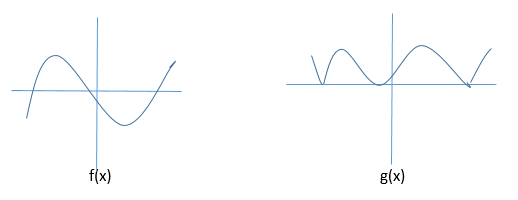The graphs of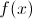and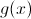are shown above. Which equation best describes the relationship betweenand?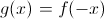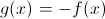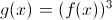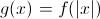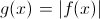Explanation: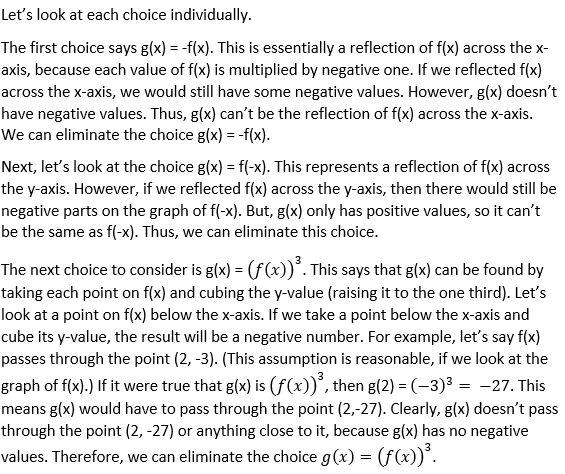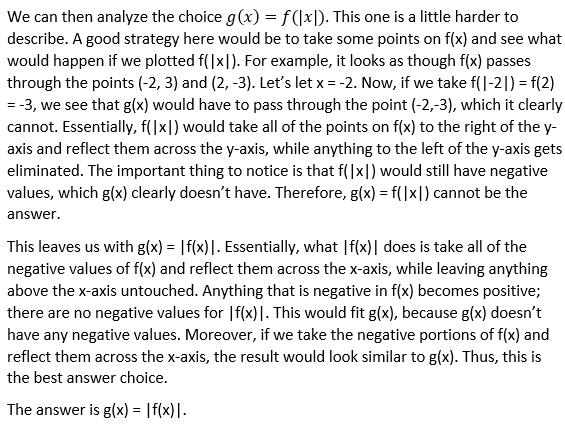### Example Question #1 : Transformation

If the point (6, 7) is reflected over the line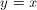and then over the x-axis, what is the resulting coordinate?

(6, 7)

(6, –7)

(7, –6)

(–6, –7)

(7, 6)

(7, –6)

Explanation:

A reflection over the lineinvolves a switching of the coordinates to get us (7, 6). A reflection over the x-axis involves a negation of the y-coordinate. Thus the resulting point is (7, –6).

### Example Question #1 : How To Find Transformation For An Analytic Geometry Equation

You are looking at a map of your town and your house is located at the coordinate (0,0). Your school is located at the point (3,4). If each coordinate distance is 1.3 miles, how far away is your school?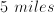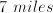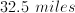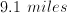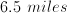Explanation:

The coordinate length between you and your school is equivalent to the hypotenuse of a right triangle with sides of 3 and 4 units: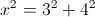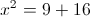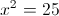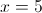The distance is 5 coordinate lengths, and each coordinate length corresponds to 1.3 miles of distance, so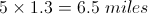### Example Question #5 : How To Find Transformation For An Analytic Geometry Equation

Line m passes through the points (–4, 3) and (2, –6). If line q is generated by reflecting m across the line y = x, then which of the  following represents the equation of q?

3x + 2y = 6

3x + 2y = 18

2x + 3y = 6

2x + 3y = –6

–2x + 3y = 6

2x + 3y = –6

Explanation:

When a point is reflected across the line y = x, the x and y coordinates are switched. In other words, the point (a, b) reflected across the line y = x would be (b, a).

Thus, if line m is reflected across the line y = x, the points that it passes through will be reflected across the line y = x. As a result, since m passes through (–4, 3) and (2, –6), when m is reflected across y = x, the points it will pass through become (3, –4) and (–6, 2).

Because line q is a reflection of line m across y = x, q must pass through the points (3, –4) and (–6, 2). We know two points on q, so if we determine the slope of q, we can then use the point-slope formula to find the equation of q.

First, let's find the slope between (3, –4) and (–6, 2) using the formula for slope between the points (x1, y1) and (x2, y2).slope = (2 – (–4))/(–6 –3)

= 6/–9 = –2/3

Next, we can use the point-slope formula to find the equation for q.

y – y1 = slope(x – x1)

y – 2 = (–2/3)(x – (–6))

Multiply both sides by 3.

3(y – 2) = –2(x + 6)

3y – 6 = –2x – 12

2x + 3y – 6 = –12

2x + 3y = –6

The answer is 2x + 3y = –6.

### Example Question #681 : Geometry

Solve each problem and decide which is the best of the choices given.

What is the amplitude of the following function?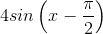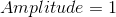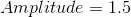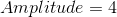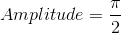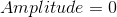Explanation:

The amplitude is always the number in front of the trig function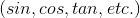.

The general equation to remember is,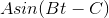whereis amplitudeis period of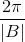andis the phase shift.

In this case it's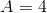.

### Example Question #1 : Transformation

The following is an equation of a circle: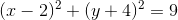If this circle is moved to the left 2 spaces and down 3 spaces, where does the center of the new circle lie?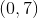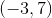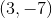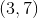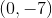Explanation:

The general formula for a circle with center (h,k) and radius r is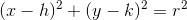.

The center of the original circle, therefore, is (2, -4).

If we move the circle to the left 2 spaces and down 3 spaces, then the center of the new circle is given by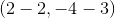or### Example Question #1 : Transformation

Let f(x) = x3 – 2x2 + x +4. If g(x) is obtained by reflecting f(x) across the y-axis, then which of the following is equal to g(x)?

–x3 – 2x2 – x – 4

–x3 + 2x2 – x + 4

–x3 – 2x2 – x + 4

x3 + 2x2 + x + 4

x3 – 2x2 – x + 4

–x3 – 2x2 – x + 4

Explanation:

In order to reflect a function across the y-axis, all of the x-coordinates of every point on that function must be multiplied by negative one. However, the y-values of each point on the function will not change. Thus, we can represent the reflection of f(x) across the y-axis as f(-x). The figure below shows a generic function (not f(x) given in the problem) that has been reflected across the y-axis, in order to offer a better visual understanding.Therefore, g(x) = f(–x).

f(x) = x3 – 2x2 + x – 4

g(x) = f(–x) = (–x)3 – 2(–x)2 + (–x) + 4

g(x) = (–1)3x3 –2(–1)2x2 – x + 4

g(x) = –x3 –2x2 –x + 4.

The answer is –x3 –2x2 –x + 4.

### Example Question #1 : Transformation

Bobby draws a circle on graph paper with a center at (2, 5) and a radius of 10.

Jenny moves Bobby's circle up 2 units and to the right 1 unit.

What is the equation of Jenny's circle?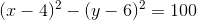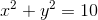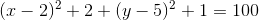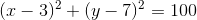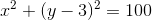Explanation:

If Jenny moves Bobby's circle up 2 units and to the right 1 unit, then the center of her circle is (3, 7). The radius remains 10.

The general equation for a circle with center (h, k) and radius r is given byFor Jenny's circle, (h, k) = (3, 7) and r=10.

Substituting these values into the general equation gives us← Previous 1

### All SAT Math Resources